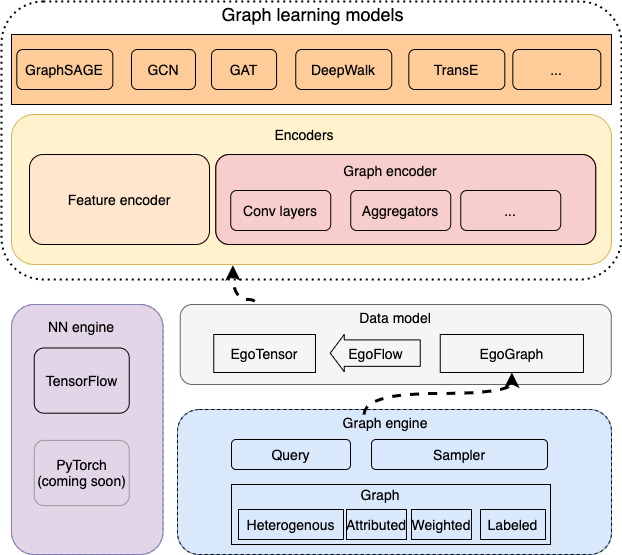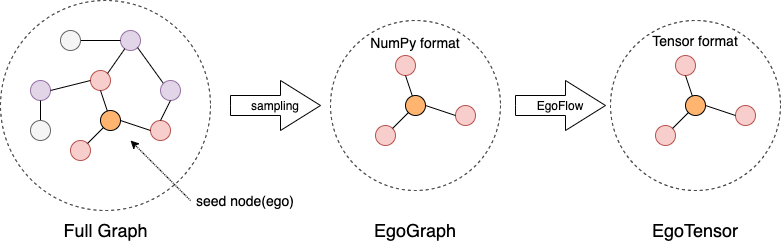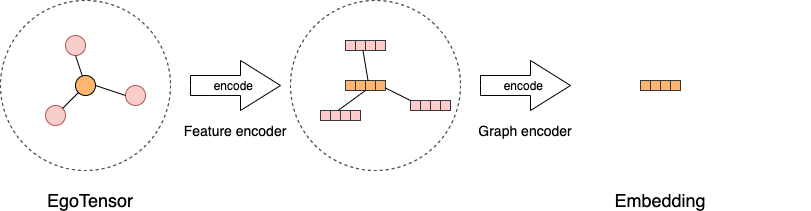# 图学习引擎#

GraphScope中的图学习引擎 (GLE) 是面向大规模图神经网络的研发和应用而设计的一款分布式框架。 它从实际问题出发，提炼和抽象了一套适合于当下图神经网络模型的编程范式， 并已经成功应用在阿里巴巴 内部的诸如搜索推荐、网络安全、知识图谱等众多场景。 GL注重可移植和可扩展，对于开发者更为友好，为了应对GNN在工业场景中的多样性和快速发展的需求。 基于GL，开发者可以实现一种GNN算法，或者面向实际场景定制化一种图算子，例如图采样。

## 图学习模型#

GLE 主要面向超大规模图神经网络的开发。它由底层的一个图引擎和上层的 算法模型构成。图引擎分布式存储图的拓扑和属性信息并提供高效的图采样查询 接口，算法模型通过调用图采样和查询接口获取子图并进行计算。

GLE 提供了一个图学习算法的统一编程框架，支持常见图学习算法的开发，包括 GNNs, 知识图谱模型，图嵌入算法等，并且和主流的深度学习算法兼容，包括TensorFlow 和PyTorch。目前我们实现了基于TensorFlow的模型，基于PyTorch的模型正在开发中。### 数据模型#

GLE 采用采样子图再计算的方式构建和训练模型。我们首先介绍一下基本的数据模型。

EgoGraph 是图学习算法编程的基本数据对象。它由一个batch的种子点或者边(称为’ego’) 以及他们的’感受野’(多跳邻居)组成。EgoGraph 由图采样和查询到的数据组成，我们实现 了常见的邻居采样、图遍历和负采样方法。### 编码器### 自定义算法#

• 指定采样模式：用图采样、查询方法采样子图并组织成 EgoGraph

我们抽象了4个基本的函数，sample_seed, positive_sample, negative_samplereceptive_fnsample_seed 用来遍历图数据产生 Nodes 或者 Edges, 然后 positve_sample 以这些 Nodes 或者 Edges 为输入产生 训练的正样本。对于无监督学习 negative_sample 产生负样本。 GNNs需要聚合邻居信息, 我们抽象了 receptive_fn 来采样邻居。 最后将 sample_seed 产生的 NodesEdges 以及采样出的邻居组成 EgoGraph

• 构建图数据流：使用 EgoFlowEgoGraph 转换为 EgoTensor

GLE 算法模型基于类似TensorFlow的深度学习引擎构建。所以采样出的 EgoGraphs 需要先转换成tensor格式 EgoTensor 才能使用。我们抽象了 EgoFlow 来封装这一转换过程。 EgoFlow 可以产生一个迭代器来进行批次训练。

• 定义编码器：使用 EgoGraph 编码器和特征编码器来编码 EgoTensor。 得到 EgoTensor 后，我们首先将原始的点、边特征用一些常见特征编码器编码成原始向量， 做为GNNs模型的特征输入。然后用图编码器处理 EgoTensor，将邻居节点特征进行汇聚并 和自身特征进行组合，得到最后的点或者边的向量。

• 编写损失函数和训练过程：选择适当的损失函数，并编写训练过程。

GLE 内置了一些常见的损失函数和优化器，并对训练过程进行了封装，同时支持单机和分布式训练。 你也可以自定义损失函数、优化器和训练过程。

### 采样#

import graphlearn as gle
g = gle.Graph()\
.node(dataset_folder + "node_table", node_type=node_type,
decoder=gle.Decoder(labeled=True,
attr_types=["float"] * 1433,
attr_delimiter=":"))\
.edge(dataset_folder + "edge_table_with_self_loop",
edge_type=(node_type, node_type, edge_type),
decoder=gle.Decoder(weighted=True), directed=False)\
.node(dataset_folder + "train_table", node_type="train",
decoder=gle.Decoder(weighted=True))\
.node(dataset_folder + "val_table", node_type="val",
decoder=gle.Decoder(weighted=True))\
.node(dataset_folder + "test_table", node_type="test",
decoder=gle.Decoder(weighted=True))


class GCN(gle.LearningBasedModel):
def __init__(self,
graph,
output_dim,
features_num,
batch_size,
categorical_attrs_desc='',
hidden_dim=16,
hops_num=2,):
self.graph = graph
self.batch_size = batch_size


GCN模型继承自基本的学习模型类 LearningBasedModel，只需要重写基类的采样， 模型构建等方法就可以完成GCN的构建。

class GCN(gle.LearningBasedModel):
# ...
def _sample_seed(self):
return self.graph.V('train').batch(self.batch_size).values()

def _positive_sample(self, t):
return gle.Edges(t.ids, self.node_type,
t.ids, self.node_type,
self.edge_type, graph=self.graph)

def _receptive_fn(self, nodes):
return self.graph.V(nodes.type, feed=nodes).alias('v') \
.outV(self.edge_type).sample().by('full').alias('v1') \
.outV(self.edge_type).sample().by('full').alias('v2') \
.emit(lambda x: gle.EgoGraph(x['v'], [ag.Layer(nodes=x['v1']), ag.Layer(nodes=x['v2'])]))


### 图数据流#

build 函数里我们使用封装的 EgoFlow 来把 EgoGraph 转换成对应的 EgoTensor, EgoFlow 包含一个数据流迭代器和若干 EgoTensor

class GCN(gle.LearningBasedModel):
def build(self):
ego_flow = gle.EgoFlow(self._sample_seed,
self._positive_sample,
self._receptive_fn,
self.src_ego_spec)
iterator = ego_flow.iterator
pos_src_ego_tensor = ego_flow.pos_src_ego_tensor
# ...


### 模型#

class GCN(gle.LearningBasedModel):
def _encoders(self):
depth = self.hops_num
feature_encoders = [gle.encoders.IdentityEncoder()] * (depth + 1)
conv_layers = []
# for input layer
conv_layers.append(gle.layers.GCNConv(self.hidden_dim))
# for hidden layer
for i in range(1, depth - 1):
conv_layers.append(gle.layers.GCNConv(self.hidden_dim))
# for output layer
conv_layers.append(gle.layers.GCNConv(self.output_dim, act=None))
encoder = gle.encoders.SparseEgoGraphEncoder(feature_encoders,
conv_layers)
return {"src": encoder, "edge": None, "dst": None}


### 损失函数和训练过程#

class GCN(gle.LearningBasedModel):
# ...
def _supervised_loss(self, emb, label):
return tf.reduce_mean(tf.nn.sparse_softmax_cross_entropy_with_logits(emb, label))

def build(self):
ego_flow = gle.EgoFlow(self._sample_seed,
self._positive_sample,
self._receptive_fn,
self.src_ego_spec,
full_graph_mode=self.full_graph_mode)
iterator = ego_flow.iterator
pos_src_ego_tensor = ego_flow.pos_src_ego_tensor
src_emb = self.encoders['src'].encode(pos_src_ego_tensor)
labels = pos_src_ego_tensor.src.labels
loss = self._supervised_loss(src_emb, labels)

return loss, iterator


def train(config, graph)
def model_fn():
return GCN(graph,
config['class_num'],
config['features_num'],
config['batch_szie'],
...)
trainer = gle.LocalTFTrainer(model_fn, epoch=200)
trainer.train()

def main():
config = {...}